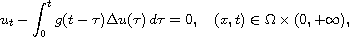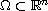Electron. J. Diff. Equ., Vol. 2012 (2012), No. 73, pp. 1-14.

### Decay results for viscoelastic diffusion equations in absence of instantaneous elasticity Mohammad Kafini

Abstract:
We study the diffusion equation in the absence of instantaneous elasticitywhere, subjected to nonlinear boundary conditions. We prove that if the relaxation function g decays exponentially, then the solutions is exponential stable.

Submitted December 18, 2011. Published May 10, 2012.
Math Subject Classifications: 35B05, 35L05, 35L15, 35L70.
Key Words: Diffusion equation; instantaneous elasticity; exponential decay; relaxation function; viscoelastic.

Show me the PDF file (219 KB), TEX file, and other files for this article.Mohammad Kafini Department of Mathematics and Statistics KFUPM, Dhahran 31261 Saudi Arabia email: mkafini@kfupm.edu.sa